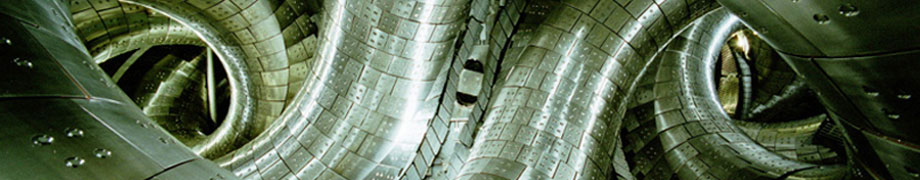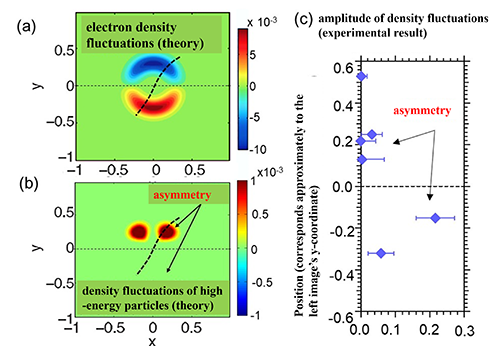August 29, 2018
Asymmetry that Appears in Plasma: Comparison between Density Measurements Based upon the Heavy Ion Beam Probe and Theoretical Research

In physics research “symmetry” is an extremely important concept. What is symmetry? For example, when we fold a star mark ✩ in half vertically through the center, the folded mark will perfectly overlap. Regarding that vertical line, there will be symmetry (that is, there will be left and right symmetry). Further, if one revolves the star mark by seventy-two degrees making the middle of the mark as the center the shape will not change. The mark will remain symmetrical even regarding the revolutions. In physics research, when the phenomenon is symmetrical, that phenomenon will be easy to understand due to an examination based upon the symmetry. Even on controlling or on understanding the properties of plasma showing complicated movements, research based upon symmetry has performed important roles. On the other hand, the phenomenon in which symmetry seems to break is an important research topic. Based upon recent advances in measurement technologies, theory, and numerical simulation technologies, we now can search for asymmetries that appear in a plasma, and we are now able to theorize regarding its qualities. Here, we will introduce research regarding asymmetries that have been discovered in the Large Helical Device (LHD).

In high-temperature plasmas, various types of oscillation occur. Based upon those oscillations, plasma density fluctuations occur. LHD plasmas take the shape of a twisted doughnut. When we measure the distribution of density fluctuations in a vertically elongated cross-section, it is expected that in that cross section density fluctuations generally become of up-down symmetry. However, it became clear that there were times when became largely of the asymmetry. Why such a large asymmetry occurs in a plasma was answered in joint research with Kyushu University. As a result of a theoretical investigation, we discovered that the effects of high energy particles that were injected in order to heat the plasma are important. According to theory, the movement of energetic particles and plasma fluctuations resonate with each other, which results in large up-down asymmetry in fluctuations of high-energy particles. We now understand that there is the possibility that this up-down asymmetry fulfills the important role in experimental observations of the asymmetry.

In order to verify this theory, we put forward our analysis of the experimental data. In the LHD, using the diagnostics called the heavy ion beam probe (below as HIBP), we are measuring the fluctuations of plasma density. In the HIBP, we inject the high-energy heavy ions from outside the plasma (for example, ions of heavy elements such a gold). When the heavy ions collide with the plasma particles, the charge number changes and, subsequently, escape to outside the plasma. The number of heavy ions that collide with the plasma depends upon the plasma density. Thus, if we carefully measure the amount of heavy ions that escaped and changed their charge number and we analyze the measurement data, we will learn the plasma density. Until now, when we analyzed HIBP measurement data we did not consider the presence of the high-energy particles. Here, as a result of our analysis that considered the density fluctuations of high-energy particles, we showed that the large asymmetry in plasma density fluctuations measured in experiments may be explained theoretically. Due to this finding, we have advanced one step in our verification of theoretical predictions. In the next experimental campaign we will advance further verification of theory by conducting experiments under various conditions.

Further, based upon this theory, interesting phenomena are foreseen from the perspective of fusion research, such that such an asymmetry of fluctuation may induce ion heating and influence the confinement of plasma. In actuality, when such an asymmetry occurred, a rise in the ion temperature has been observed. Regarding cause and effect there is as yet no conclusion. However, we are moving forward in preparing an experiment that will examine this issue.Image: Up-down asymmetry in electron density fluctuations

Caption: (a) and (b) show results of a prediction of how based upon a theory regarding how the density of electrons and high energy particles fluctuate in a cross-section of plasma. The plasma center is at the point (x, y) = (0, 0), the vertical axis (y) shows the position above and below from the plasma center, and the horizontal axis (x) shows inner and outer sides from the center. The part where the density decreases is shown in from water color to blue, and the area where the density increases is shown in from yellow to red. The deeper the color becomes the larger the amplitudes become. Changes in the electron density (fluctuations) indicate that growth and reduction are reversed, but above and below are symmetrical. Conversely, the variations (fluctuations) of high-energy particle density are up-down asymmetric. (Sasaki Masahiro, Kyushu University)
(c) shows the LHD experimental results of the magnitude of the density fluctuations at the position indicated by the thick dotted line in the left image. The plasma center corresponds to the ordinate axis of 0.0 (horizontal dotted line). The magnitude of the amplitude of density fluctuation (the horizontal axis) is larger below the center, indicating the up-down asymmetry.
By taking account of density distribution of high-energy particles predicted by theory upon analyzing the measurement data, in the region of y > 0 the negative contribution of density fluctuations (a) is cancelled by the positive contribution of density fluctuations of high-energy particles (b), which results in the small amplitude of density fluctuations of plasma. From this, we have become able to explain theoretically the asymmetry (c) that was observed in the experiment.